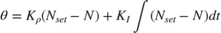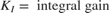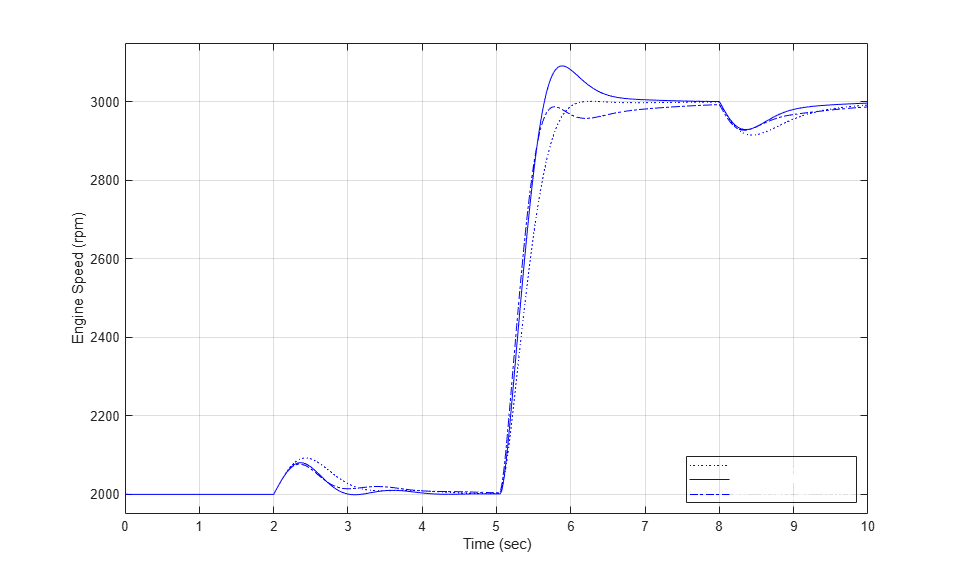# Engine Timing Model with Closed Loop Control

This example shows how to enhance a version of the open-loop engine model described in Modeling Engine Timing Using Triggered Subsystems. This model, sldemo_enginewc, contains a closed-loop and shows the flexibility and extensibility of Simulink® models. In this enhanced model, the objective of the controller is to regulate engine speed with a fast throttle actuator, such that changes in load torque have minimal effect. This is easily accomplished in Simulink by adding a discrete-time PI controller to the engine model.

### Closed-Loop Model

We chose a control law which uses proportional plus integral (PI) control. The integrator is needed to adjust the steady-state throttle as the operating point changes, and the proportional term compensates for phase lag introduced by the integrator.

Equation 1### Running the Simulation

Press the "Play" button on the model toolbar to run the simulation.

• Note: The model logs relevant data to MATLAB workspace in a structure called sldemo_enginewc_output. Logged signals have a blue indicator. Read more about Signal Logging in Simulink Help.Figure 1: Closed-loop engine model and simulation results

In this model we employ a discrete-time controller, which is suitable for microprocessor implementation. The integral term in Equation 1 must thus be realized with a discrete-time approximation. As is typical in the industry, the controller execution is synchronized with the engine's crankshaft rotation. The controller is embedded in a triggered subsystem that is triggered by the valve timing signal described above.

The detailed construction of the 'Controller' subsystem is illustrated in Figure 2. Of note is the use of the 'PID Controller' block. This block implements a proportional-integral control system in discrete time. Note the setting for sample time set (internally) at -1. This indicates that the block inherits its sample time, in this case executing each time the subsystem is triggered. The key component that makes this a triggered subsystem is the 'Trigger' block shown at the bottom of Figure 2. Any subsystem can be converted to a triggered subsystem by dragging a copy of this block into the subsystem diagram from the Simulink Connections library.Figure 2: Speed controller subsystem

### Results

Typical simulation results are shown in Figure 3. The speed set point steps from 2000 rpm to 3000 rpm at t = 5 sec. The torque disturbances are identical to those used in sldemo_engine, the open-loop model. Note the quick transient response, with zero steady-state error. Several alternative controller tunings (Ki and Kp) are shown. These can be adjusted by the user at MATLAB command line. This allows the engineer to understand the relative effects of parameter variations.Figure 3: Typical simulation results

### Closing Model

Close the model. Clear logged data.

### Conclusions

The ability to model nonlinear, complex systems, such as the engine model described here, is one of Simulink's key features. The power of the simulation is evident in the presentation of the models above. Simulink retains model fidelity, including precisely timed cylinder intake events, which is critical in creating a model of this type. The complete speed control system shows the flexibility of Simulink. In particular, the Simulink modeling approaches allow rapid prototyping of an interrupt-driven engine speed controller.

### References

 P.R. Crossley and J.A. Cook, IEEE® International Conference 'Control 91', Conference Publication 332, vol. 2, pp. 921-925, 25-28 March, 1991, Edinburgh, U.K.

 The Simulink Model. Developed by Ken Butts, Ford Motor Company®. Modified by Paul Barnard, Ted Liefeld and Stan Quinn, MathWorks®, 1994-7.

 J. J. Moskwa and J. K. Hedrick, "Automotive Engine Modeling for Real Time Control Application," Proc.1987 ACC, pp. 341-346.

 B. K. Powell and J. A. Cook, "Nonlinear Low Frequency Phenomenological Engine Modeling and Analysis," Proc. 1987 ACC, pp. 332-340.

 R. W. Weeks and J. J. Moskwa, "Automotive Engine Modeling for Real-Time Control Using Matlab/Simulink," 1995 SAE Intl. Cong. paper 950417.